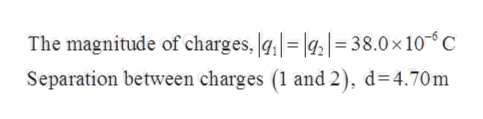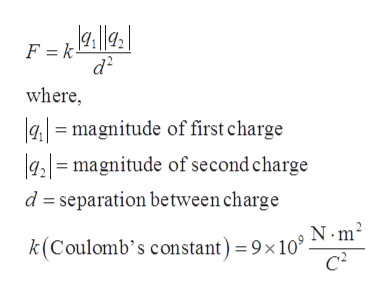Question
189 views

In Figure (a) particles 1 and 2 have charge 38.0 μC each and are held at separation distance d = 4.70 m.

(a) What is the magnitude of the electrostatic force on particle 1 due to particle 2? In Figure (b), particle 3 of charge 38.0 μC is positioned so as to complete an equilateral triangle.

(b) What is the magnitude of the net electrostatic force on particle 1 due to particles 2 and 3?

check_circle

Step 1

Given,help_outlineImage TranscriptioncloseThe magnitude of charges, = |g|= 38.0 x 10 C Separation between charges (1 and 2), d 4.70m fullscreen
Step 2

From Coulomb’s law, magnitude of force between two charge,help_outlineImage TranscriptioncloseF =kl|g k - d2 where amagnitude of first charge g,=magnitude of second charge d separation between charge N m2 k(Coulomb's constant) = 9 x 10' c fullscreen
Step 3

Part (a)

Since, both charges 1 and 2 have equal sign, the...

### Want to see the full answer?

See Solution

#### Want to see this answer and more?

Solutions are written by subject experts who are available 24/7. Questions are typically answered within 1 hour.*

See Solution
*Response times may vary by subject and question.
Tagged in

### Electric Charges and Fields